# Blending/Overlaying images¶

## Introduction¶

Most augmenters in the library affect images in uniform ways per image. Sometimes one might not want that and instead desires more localized effects (e.g. change the color of some image regions, while keeping the others unchanged) or wants to keep a fraction of the old image (e.g. blur the image and mix in a bit of the unblurred image). Blending augmenters are intended for these use cases. They either mix two images using a constant alpha factor or using a pixel-wise mask. Below image shows examples.

```# First row
iaa.BlendAlpha(
(0.0, 1.0),
foreground=iaa.MedianBlur(11),
per_channel=True
)

# Second row
iaa.BlendAlphaSimplexNoise(
foreground=iaa.EdgeDetect(1.0),
per_channel=False
)

# Third row
iaa.BlendAlphaSimplexNoise(
foreground=iaa.EdgeDetect(1.0),
background=iaa.LinearContrast((0.5, 2.0)),
per_channel=0.5
)

# Forth row
iaa.BlendAlphaFrequencyNoise(
foreground=iaa.Affine(
rotate=(-10, 10),
translate_px={"x": (-4, 4), "y": (-4, 4)}
),
per_channel=0.5
)

# Fifth row
iaa.BlendAlphaSimplexNoise(
foreground=iaa.BlendAlphaSimplexNoise(
foreground=iaa.EdgeDetect(1.0),
background=iaa.LinearContrast((0.5, 2.0)),
per_channel=True
),
background=iaa.BlendAlphaFrequencyNoise(
exponent=(-2.5, -1.0),
foreground=iaa.Affine(
rotate=(-10, 10),
translate_px={"x": (-4, 4), "y": (-4, 4)}
),
per_channel=True
),
per_channel=True,
aggregation_method="max",
sigmoid=False
)
```

## Imagewise Constant Alphas Values¶

The augmenter `imgaug.augmenters.blend.BlendAlpha` allows to mix the results of two augmentation branches using an alpha factor that is constant throughout the whole image, i.e. it follows roughly `I_blend = alpha * I_fg + (1 - alpha) * I_bg` per image, where `I_fg` is the image from the foreground branch and `I_bg` is the image from the background branch. Often, the first branch will be an augmented version of the image and the second branch will be the identity function, leading to a blend of augmented and unaugmented image. The background branch can also contain non-identity augmenters, leading to a blend of two distinct augmentation effects.

`imgaug.augmenters.blend.BlendAlpha` is already built into some augmenters as a parameter, e.g. into `imgaug.augmenters.convolutional.EdgeDetect`.

The below example code generates images that are a blend between `imgaug.augmenters.convolutional.Sharpen` and `imgaug.augmenters.arithmetic.CoarseDropout`. Notice how the sharpening does not affect the black rectangles from dropout, as the two augmenters are both applied to the original images and merely blended.

```import imgaug as ia
from imgaug import augmenters as iaa

ia.seed(1)

# Example batch of images.
# The array has shape (8, 128, 128, 3) and dtype uint8.
images = np.array(
[ia.quokka(size=(128, 128)) for _ in range(8)],
dtype=np.uint8
)

seq = iaa.BlendAlpha(
factor=(0.2, 0.8),
foreground=iaa.Sharpen(1.0, lightness=2),
background=iaa.CoarseDropout(p=0.1, size_px=8)
)

images_aug = seq(images=images)
```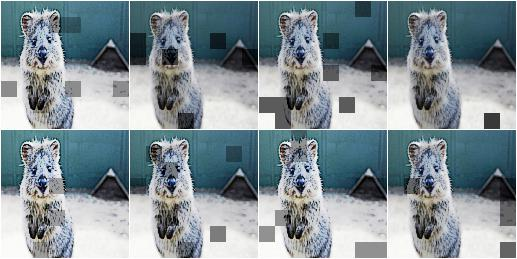Mixing `imgaug.augmenters.convolutional.Sharpen` and `imgaug.augmenters.arithmetic.CoarseDropout` via `imgaug.augmenters.blend.BlendAlpha`. The resulting effect is very different from executing them in sequence.

Similar to other augmenters, `imgaug.augmenters.blend.BlendAlpha` supports a `per_channel` mode, in which it samples blending strengths for each channel independently. As a result, some channels may show more from the foreground (or background) branch’s outputs than other channels. This can lead to visible color effects. The following example is the same as the one above, only `per_channel` was activated.

```iaa.BlendAlpha(..., per_channel=True)
```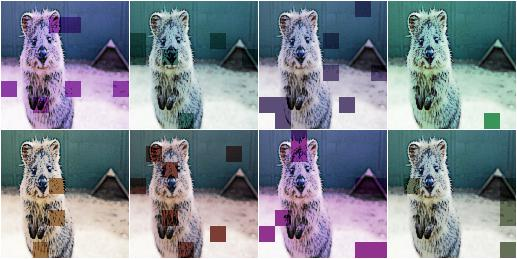Mixing `imgaug.augmenters.convolutional.Sharpen` and `imgaug.augmenters.arithmetic.CoarseDropout` via `imgaug.augmenters.blend.BlendAlpha` and `per_channel` set to `True`.

`imgaug.augmenters.blend.BlendAlpha` can also be used with augmenters that change the position of pixels, leading to “ghost” images. (This should not be done when also augmenting keypoints, as their position becomes unclear.)

```seq = iaa.BlendAlpha(
factor=(0.2, 0.8),
foreground=iaa.Affine(rotate=(-20, 20)),
per_channel=True
)
```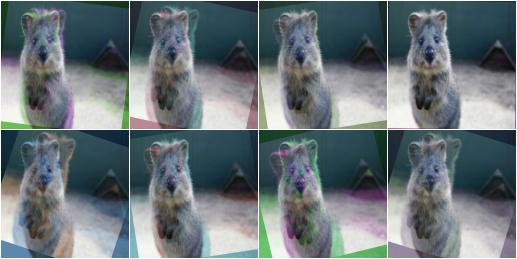Mixing original images with their rotated version. Some channels are more visibly rotated than others.

## BlendAlphaSimplexNoise¶

`imgaug.augmenters.blend.BlendAlpha` uses a constant blending factor per image (or per channel). This limits its possibilities. Often, a more localized factor is desired to create unusual patterns. `imgaug.augmenters.blend.BlendAlphaSimplexNoise` is an augmenter that does that. It generates continuous masks following simplex noise and uses them to perform local blending. The following example shows a combination of `imgaug.augmenters.blend.BlendAlphaSimplexNoise` and `imgaug.augmenters.arithmetic.Multiply` (with `per_channel=True`) that creates blobs of various colors in the image.

```import imgaug as ia
from imgaug import augmenters as iaa

ia.seed(1)

# Example batch of images.
# The array has shape (8, 128, 128, 3) and dtype uint8.
images = np.array(
[ia.quokka(size=(128, 128)) for _ in range(8)],
dtype=np.uint8
)

seq = iaa.SimplexNoiseAlpha(
foreground=iaa.Multiply(iap.Choice([0.5, 1.5]), per_channel=True)
)

images_aug = seq(images=images)
```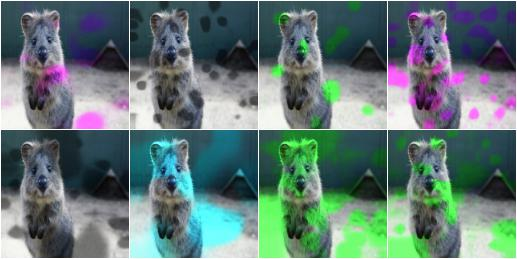Mixing original images with their versions modified by `imgaug.augmenters.arithmetic.Multiply` (with `per_channel` set to `True`). Simplex noise masks are used for the blending process, leading to blobby patterns.

`imgaug.augmenters.blend.BlendAlphaSimplexNoise` also supports `per_channel=True`, leading to unique noise masks sampled per channel. The following example shows the combination of `imgaug.augmenters.blend.BlendAlphaSimplexNoise` (with `per_channel=True`) and `imgaug.augmenters.convolutional.EdgeDetect`. Even though `imgaug.augmenters.convolutional.EdgeDetect` usually generates black and white images (white=edges, black=everything else), here the combination leads to strong color effects as the channel-wise noise masks only blend EdgeDetect’s result for some channels.

```seq = iaa.BlendAlphaSimplexNoise(
foreground=iaa.EdgeDetect(1.0),
per_channel=True
)
```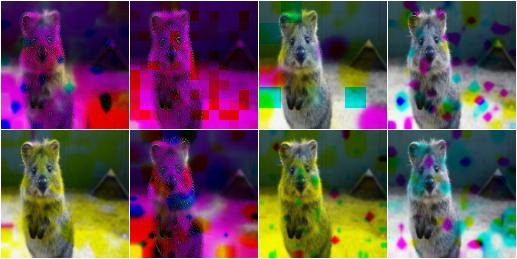Blending images via simplex noise can lead to unexpected but diverse patterns when `per_channel` is set to `True`. Here, a mixture of original images with `EdgeDetect(1.0)` is used.

`imgaug.augmenters.blend.BlendAlphaSimplexNoise` uses continuous noise masks (2d arrays with values in the range [0.0, 1.0]) to blend images. The below image shows examples of 64x64 noise masks generated by `imgaug.augmenters.blend.BlendAlphaSimplexNoise` with default settings. Values close to `1.0` (white) indicate that pixel colors will be taken from the first image source, while `0.0` (black) values indicate that pixel colors will be taken from the second image source. (Often only one image source will be given in the form of augmenters and the second will fall back to the original images fed into `imgaug.augmenters.blend.BlendAlphaSimplexNoise`.)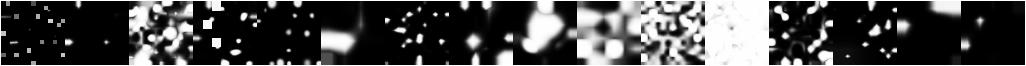Examples of noise masks generated by `imgaug.augmenters.blend.BlendAlphaSimplexNoise` using default settings.

`imgaug.augmenters.blend.BlendAlphaSimplexNoise` generates its noise masks in low resolution images and then upscales the masks to the size of the input images. During upscaling it usually uses nearest neighbour interpolation (`nearest`), linear interpolation (`linear`) or cubic interpolation (`cubic`). Nearest neighbour interpolation leads to noise maps with rectangular blobs. The below example shows noise maps generated when only using nearest neighbour interpolation.

```seq = iaa.BlendAlphaSimplexNoise(
...,
upscale_method="nearest"
)
```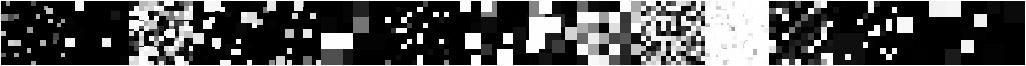Examples of noise masks generated by `imgaug.augmenters.blend.BlendAlphaSimplexNoise` when restricting the upscaling method to `nearest`.

Similarly, the following example shows noise maps generated when only using linear interpolation.

```seq = iaa.BlendAlphaSimplexNoise(
...,
upscale_method="linear"
)
```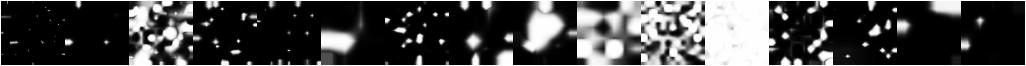Examples of noise masks generated by `imgaug.augmenters.blend.BlendAlphaSimplexNoise` when restricting the upscaling method to `linear`.

## FrequencyNoiseAlpha¶

`imgaug.augmenters.blend.BlendAlphaFrequencyNoise` is mostly identical to `imgaug.augmenters.blend.BlendAlphaSimplexNoise`. In contrast to `imgaug.augmenters.blend.BlendAlphaSimplexNoise` it uses a different sampling process to generate the blend masks. The process is based on starting with random frequencies, weighting them with a random exponent and then transforming from frequency domain to spatial domain. When using a low exponent value this leads to large, smooth blobs. Slightly higher exponents lead to cloudy patterns. High exponent values lead to recurring, small patterns. The below example shows the usage of `imgaug.augmenters.blend.BlendAlphaFrequencyNoise`.

```import imgaug as ia
from imgaug import augmenters as iaa
from imgaug import parameters as iap

ia.seed(1)

# Example batch of images.
# The array has shape (8, 64, 64, 3) and dtype uint8.
images = np.array(
[ia.quokka(size=(128, 128)) for _ in range(8)],
dtype=np.uint8
)

seq = iaa.BlendAlphaFrequencyNoise(
foreground=iaa.Multiply(iap.Choice([0.5, 1.5]), per_channel=True)
)

images_aug = seq.augment_images(images)
```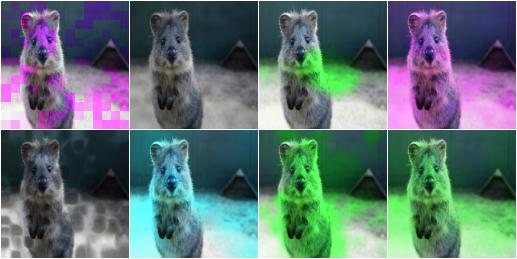Mixing original images with their versions modified by `imgaug.augmenters.arithmetic.Multiply` (with `per_channel` set to `True`). Frequency noise masks are used for the blending process, leading to blobby patterns.

Similarly to simplex noise, `imgaug.augmenters.blend.BlendAlphaFrequencyNoise` also supports `per_channel=True`, leading to different noise maps per image channel.

```seq = iaa.BlendAlphaFrequencyNoise(
foreground=iaa.EdgeDetect(1.0),
per_channel=True
)
```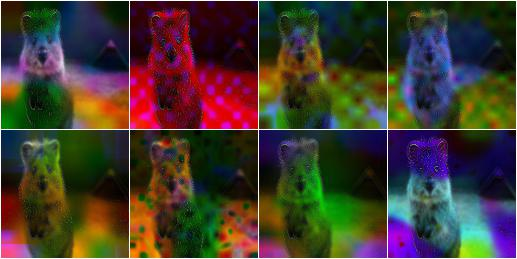Blending images via frequency noise can lead to unexpected but diverse patterns when `per_channel` is set to `True`. Here, a mixture of original images with `imgaug.augmenters.convolutional.EdgeDetect(1.0)` is used.

The below image shows random example noise masks generated by `imgaug.augmenters.blend.BlendAlphaFrequencyNoise` with default settings.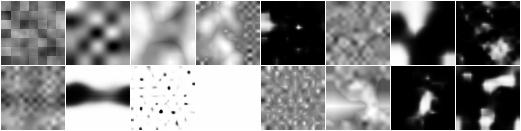Examples of noise masks generated by `imgaug.augmenters.blend.FrequencyNoiseAlpha` using default settings.

The following image shows the effects of varying `exponent` between `-4.0` and `4.0`. To show these effects more clearly, a few features of `imgaug.augmenters.blend.BlendAlphaFrequencyNoise` were deactivated (e.g. multiple iterations). In the code, `E` is the value of the exponent (e.g. `E=-2.0`).

```seq = iaa.BlendAlphaFrequencyNoise(
exponent=E,
foreground=iaa.Multiply(iap.Choice([0.5, 1.5]), per_channel=True),
size_px_max=32,
upscale_method="linear",
iterations=1,
sigmoid=False
)
```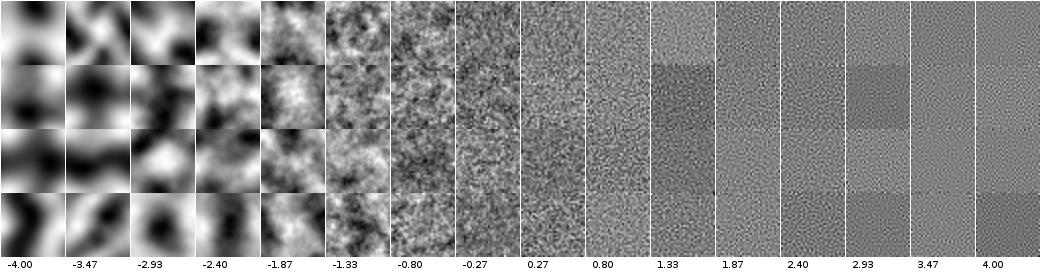Examples of noise masks generated by `imgaug.augmenters.blend.BlendAlphaFrequencyNoise` using default settings with varying exponents.

Similarly to `imgaug.augmenters.blend.BlendAlphaSimplexNoise`, `imgaug.augmenters.blend.BlendAlphaFrequencyNoise` also generates the noise masks as low resolution versions and then upscales them to the full image size. The following images show the usage of nearest neighbour interpolation (`upscale_method="nearest"`) and linear interpolation (`upscale_method="linear"`).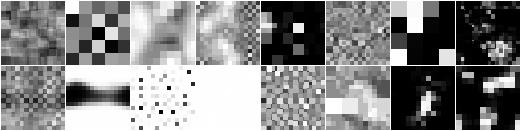Examples of noise masks generated by `imgaug.augmenters.blend.BlendAlphaFrequencyNoise` when restricting the upscaling method to `nearest`.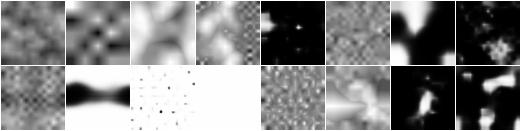Examples of noise masks generated by `imgaug.augmenters.blend.BlendAlphaFrequencyNoise` when restricting the upscaling method to `linear`.

## IterativeNoiseAggregator¶

Both `imgaug.augmenters.blend.BlendAlphaSimplexNoise` and `imgaug.augmenters.blend.BlendAlphaFrequencyNoise` wrap around `imgaug.parameters.IterativeNoiseAggregator`, a component to generate noise masks in multiple iterations. It has parameters for the number of iterations (1 to N) and for the aggregation methods, which controls how the noise masks from the different iterations are to be combined. Valid aggregation methods are `"min"`, `"avg"` and `"max"`, where `min` takes the minimum over all iteration’s masks, `max` the maxmimum and `avg` the average. As a result, masks generated with method `min` tend to be close to 0.0 (mostly black values), those generated with `max` close to `1.0` and `avg` converges towards `0.5`. (`0.0` means that the results of the second image dominate the final image, so in many cases the original images before the augmenter). The following image shows the effects of changing the number of iterations when combining `imgaug.parameters.FrequencyNoise` with `imgaug.parameters.IterativeNoiseAggregator`.

```# This is how the iterations would be changed for BlendAlphaFrequencyNoise.
# (Same for BlendAlphaSimplexNoise.)
seq = iaa.BlendAlphaFrequencyNoise(
...,
iterations=N
)
```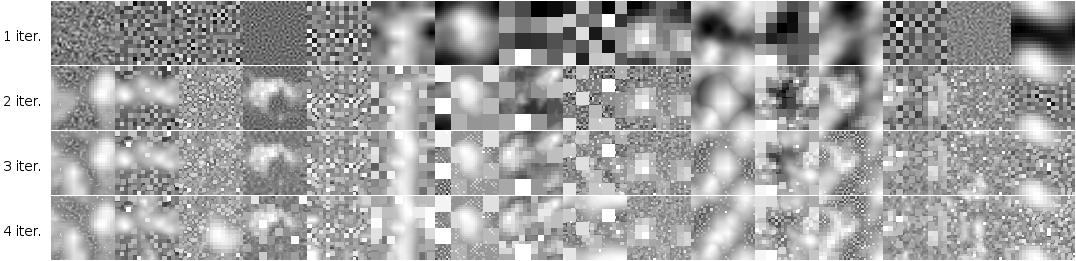Examples of varying the number of iterations in `imgaug.parameters.IterativeNoiseAggregator` (here in combination with `imgaug.parameters.FrequencyNoise`).

The following image shows the effects of changing the aggregation mode (with varying iterations).

```# This is how the iterations and aggregation method would be changed for
# BlendAlphaFrequencyNoise. (Same for BlendAlphaSimplexNoise.)
seq = iaa.BlendAlphaFrequencyNoise(
...,
iterations=N,
aggregation_method=M
)
```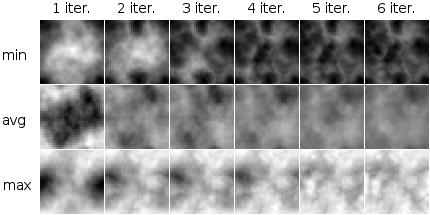Examples of varying the aggregation method and iterations in `imgaug.parameters.IterativeNoiseAggregator` (here in combination with `imgaug.parameters.FrequencyNoise`).

## Sigmoid¶

Generated noise masks can often end up having many values around 0.5, especially when running `imgaug.parameters.IterativeNoiseAggregator` with many iterations and aggregation method `avg`. This can be undesired. `imgaug.parameters.Sigmoid` is a method to compensate that. It applies a sigmoid function to the noise masks, forcing the values to mostly lie close to 0.0 or 1.0 and only rarely in between. This can lead to blobs of values close to 1.0 (“use only colors from images coming from source A”), surrounded by blobs with values close to 0.0 (“use only colors from images coming from source B”). This is similar to taking either from one image source (per pixel) or the other, but usually not both. Sigmoid is integrated into both class:imgaug.augmenters.blend.BlendAlphaSimplexNoise and `imgaug.augmenters.blend.BlendAlphaFrequencyNoise`. It can be dynamically activated/deactivated and has a threshold parameter that controls how aggressive and pushes the noise values towards 1.0.

```# This is how the Sigmoid would be activated/deactivated for
# BlendAlphaFrequencyNoise (same for BlendAlphaSimplexNoise). P is the
# probability of the Sigmoid being activated (can be True/False), T is the
# threshold (sane values are usually around -10 to +10, can be a
# tuple, e.g. sigmoid_thresh=(-10, 10), to indicate a uniform range).
seq = iaa.BlendAlphaFrequencyNoise(
...,
sigmoid=P,
sigmoid_thresh=T
)
```

The below image shows the effects of applying `imgaug.parameters.Sigmoid` to noise masks generated by `imgaug.parameters.FrequencyNoise`.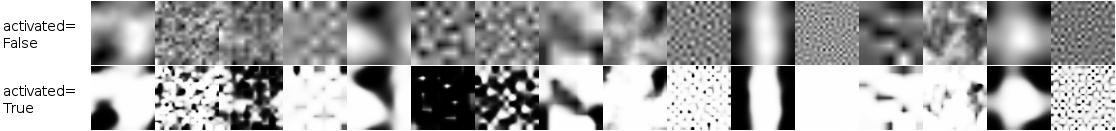Examples of noise maps without and with activated `imgaug.parameters.Sigmoid` (noise maps here from `imgaug.parameters.FrequencyNoise`).

The below image shows the effects of varying the sigmoid’s threshold. Lower values place the threshold further to the “left” (lower x values), leading to more x-values being above the threshold values, leading to more 1.0s in the noise masks.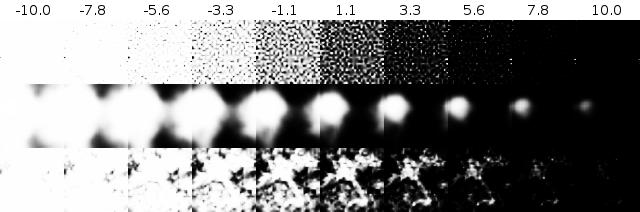Examples of varying the `imgaug.parameters.Sigmoid` threshold from `-10.0` to `10.0`.